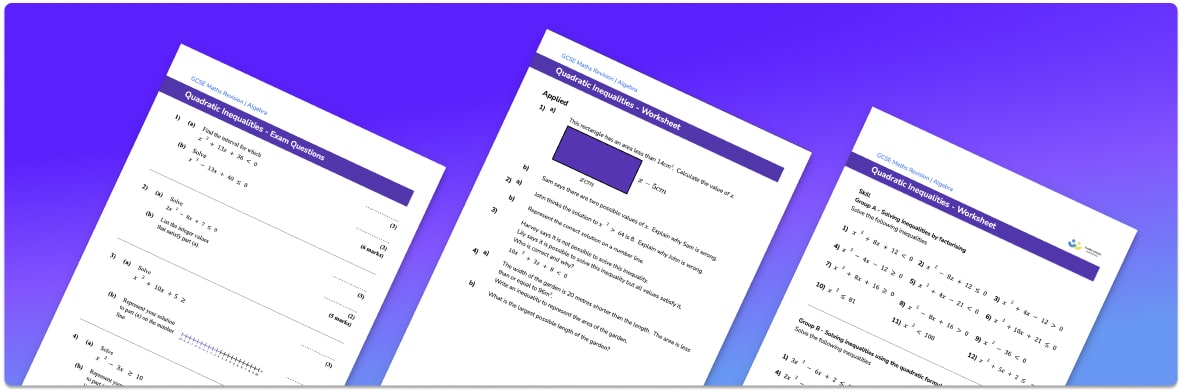• Section 1 of the solving quadratic inequalities worksheet contains 48 skills-based solving quadratic inequalities questions, in 3 groups to support differentiation
• Section 2 contains 4 applied solving quadratic inequalities questions with a mix of worded problems and deeper problem solving questions
• Section 3 contains 4 foundation and higher level GCSE exam style solving quadratic inequalities questions
• Section 3 contains 3 foundation and higher level GCSE exam style questions on solving quadratic inequalities
• Answers and a mark scheme for all quadratic inequalities questions are provided
• Questions follow variation theory with plenty of opportunities for students to work independently at their own level
• All questions created by fully qualified expert secondary maths teachers
• Suitable for GCSE maths revision for AQA, OCR and Edexcel exam boards

• This field is for validation purposes and should be left unchanged.

You can unsubscribe at any time (each email we send will contain an easy way to unsubscribe). To find out more about how we use your data, see our privacy policy.

### Solving quadratic inequalities at a glance

Quadratic inequalities are inequalities that involve a squared term. As with linear inequalities, there are four types: greater than >, less than <, greater than or equal to ≥ and less than or equal to ≤.

To solve a quadratic inequality, we first write it as a quadratic equation by changing the inequality sign to an equals sign. We can then solve the quadratic equation either by factorising or using the quadratic formula. This tells us the roots of the equation, i.e. the values for which the expression is equal to 0.

Once we have the roots of the equation, we can sketch the quadratic function – this will be a parabola. We need to look for the x values which satisfy the inequality – if the inequality is > 0 then we are looking for the x values for which the graph is above the x axis and if it is < 0 then we are looking for the x values where the graph is below the x axis.

Looking forward, students can progress with more inequalities worksheets, to additional algebra worksheets, for example a factorising worksheet or simultaneous equations worksheet.For more teaching and learning support on Algebra our GCSE maths lessons provide step by step support for all GCSE maths concepts.

## Related worksheets

Inequalities On A Number Line Worksheet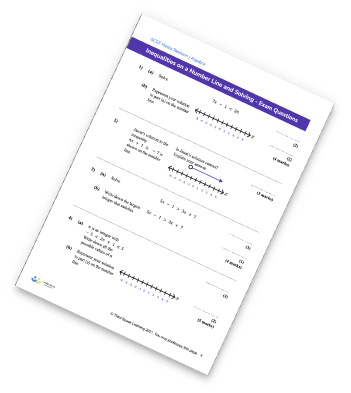Inequalities Worksheet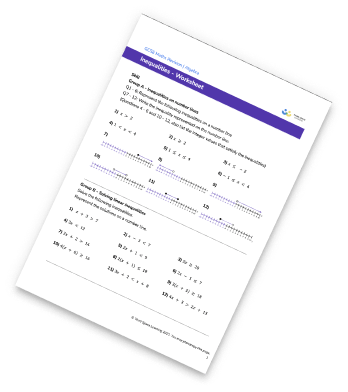Inverse Functions Worksheet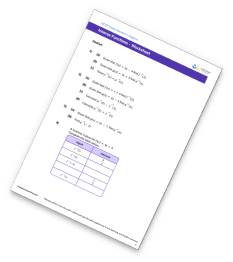Composite and Inverse Functions Worksheet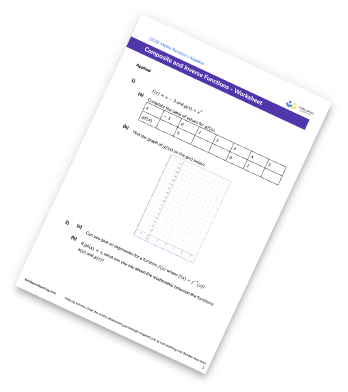## Do you have KS4 students who need more focused attention to succeed at GCSE?There will be students in your class who require individual attention to help them succeed in their maths GCSEs. In a class of 30, it’s not always easy to provide.

Help your students feel confident with exam-style questions and the strategies they’ll need to answer them correctly with our dedicated GCSE maths revision programme.

Lessons are selected to provide support where each student needs it most, and specially-trained GCSE maths tutors adapt the pitch and pace of each lesson. This ensures a personalised revision programme that raises grades and boosts confidence.

Find out more